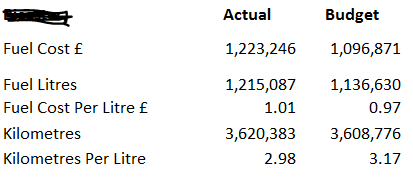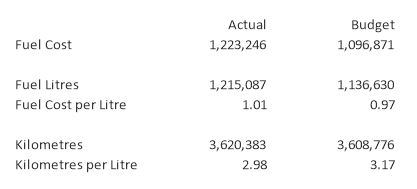# New to QlikView

Discussion board where members can get started with QlikView.

Highlighted
New Contributor II

## Calculated Dimension values in a table

Hi,

Can anyone help?

I am fairly new to QlikView. I want to create a table like this below similar to what I have in Excel:I have created a pivot table. Fuel Cost £, Fuel Litres and Kilometres are values in a KPI dimension field. Actual and Budget are calculated expressions with set analysis  - e.g. sum({<KPI = {[Fuel Cost £],[Fuel Litres],[Kilometres]},[Actual Budget]= {Actual} >} Amount).

How do I create Fuel Cost Per Litre £ so that it appears below the Fuel Litres row?

Am I doing this wrong - should I be using another type of table ?

Mel

Tags (4)
1 Solution

Accepted SolutionsMVP & Luminary

## Re: Calculated Dimension values in a table

Yes, it could work.  Enable "Partial Sums" on the chart on the Presentation tab to get Totals.  You will then need to modify each expression to use a slightly different formula to calculate the total cell. The Dimensionality() function can be used to detect the Total cell,. Dimensionality()=0 for the total.  So for example:

if(dimensionality() > 0

,sum({<KPI={'Fuel Cost'}>}Amount)

,sum({<KPI={'Fuel Cost'}, [Actual Budget]={'Actual'}>}Amount) - sum({<KPI={'Fuel Cost'}, [Actual Budget]={'Budget'}>}Amount)

)Updated example attached.

-Rob

http://qlikviewcookbook.com

http://www.easyqlik.com

3 RepliesMVP & Luminary

## Re: Calculated Dimension values in a table

Here's the approach I would recommend.

1. Pivot Table with single Dimension: [Actual Budget]

2. Separate expression for each line, e.g.;  sum({<KPI={'Fuel Cost'}>}Amount)

3. Drag the Dimension column to the upper right horizontal.

4. Drag the expressions to the left vertical.

The result can look like this:You can add some "blank" expressions to create separator lines . See attached qvw example.

-Rob

http://qlikviewcookbook.com

http://www.easyqlik.com

New Contributor II

## Re: Calculated Dimension values in a table

Hi, Rob.

Wow, that's really good.

I have a follow-on question if you don't mind - if you want to show the difference between Actual and Budget as a third column would your solution still work ?

Thankyou,

MelMVP & Luminary

## Re: Calculated Dimension values in a table

Yes, it could work.  Enable "Partial Sums" on the chart on the Presentation tab to get Totals.  You will then need to modify each expression to use a slightly different formula to calculate the total cell. The Dimensionality() function can be used to detect the Total cell,. Dimensionality()=0 for the total.  So for example:

if(dimensionality() > 0

,sum({<KPI={'Fuel Cost'}>}Amount)

,sum({<KPI={'Fuel Cost'}, [Actual Budget]={'Actual'}>}Amount) - sum({<KPI={'Fuel Cost'}, [Actual Budget]={'Budget'}>}Amount)

)Updated example attached.

-Rob